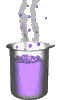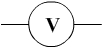Science class online
Chemistry and physics lessons

# Electricity

Free online electricity lessons for elementary school,  middle school and high school.Science class Chemistry Electricity Optics Mechanics Electricity lessons Electrical components -Two-terminal electronic components - Basic electrical components - Diodes - What's a resistor ? - How to determine a resistor value ? - Resistors effects in circuits - How to use a resistor ? - Characteristic curve of a resistor How to build simple circuits and draw diagrams - How to build a basic electrical circuit - How to draw diagrams of electric circuits The electric current - Conductors and insulators - Direction of electric current in a circuit - The dangers of electricity - Current intensity - units how to measure current ? The Voltage - The voltage and its units - How to measure a voltage ? - Voltage in open and closed circuits - Rated current and voltage for a lamp Alternating voltage and current - Alternating currents effects on led - What are alternating current and voltage ? - Periodic alternating voltage and its properties - Oscilloscope - Oscillogram - Frequency - Measuring RMS voltage with a voltmeter Serie circuits - What's a serie circuit ? - Series circuit properties - Short circuit in  series circuit - Current law in series - circuits - Voltage law in series circuits Parallel circuits - What's a parallel circuit ? - Some parallel circuits properties - Short circuits in parallel circuits - Nodes and branches in parallel circuits - Current laws in parallel circuits - Voltage laws in parallel circuits Laws of electricity - Ohm's law - Current laws in series circuits - Voltage laws in series circuits - Current laws in parallel circuits - Voltage laws in parallel circuits Generating electricity - Voltage for coil wires What is an alternator ? - Alternating currents and voltages - Generating electricity in power plants Electric power and energy - Electric power and power rating - Electric power consumption by an electrical device - Relationship between - Electric power and energy

 _____________________________________________________   _____________________________________________________

## The Voltage

### How to measure a voltage

 1) Multimeter and voltmeter The voltage is measured using a voltmeter whose symbol is:The multimeter can measure a current but it can also measure a voltage. In this case we use the voltmeter mode.     2) How to connect a multimeter in voltmeter mode ? - Terminals: We use the COM terminal and a voltage terminal (V) - The sense of connection: The COM terminal is closest to the negative terminal and the voltage terminal closest to the positive terminal of the electric generator. - The insertion in the circuit: The multimeter is connected in parallel (The multimeter is connected to the component's terminals for which we want to know the voltage). ______________________________________ ______________________________________ 3) How to choose the range for multimeter in voltmeter mode ? Definition: The chosen range corresponds to the highest voltage that the voltmeter can measure. The range used should not be smaller than the measured voltage: the measure couldn't be made and the voltmeter may be damaged. The range should not be too great otherwise the measurement looses its accuracy. Method: We must first use the highest range for an approximation of the voltage and then choose the closest (but higher) to obtain a more accurate measurement. ______________________________________ ______________________________________
____________________________________

____________________________________